# Are you ready for Mesh-Free CFD?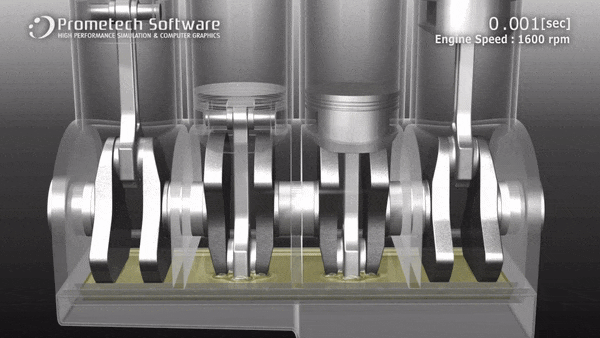## Computational Fluid Dynamic Analysis do not always require mesh creation - Introducing The Particle Method

### See the most recent Webinar Replay

A particle method is a is a method for fluid simulation that expresses fluid as groups of particles. We can simulate the fluid behavior with the particle method without needing to generate a mesh because the particles are calculation points and are able to move flexibly.

By example, below is a sloshing fluid in a container. If the container is oscillated by movement, the fluid is also oscillated.

Traditional methods can simulate fluid motion of small amplitude: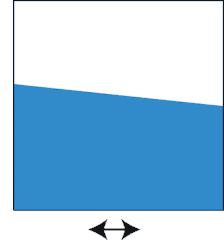See the new ANSYS Workbench Plug in here

However, it is very difficult for them to simulate large amplitude motion shown in here because of the large deformation, splitting and coalescence of fluid. If we apply traditional methods to the phenomenon, we need to carry out very complicated and advanced procedures. Besides, it will take a long time for pre-process and for the computations.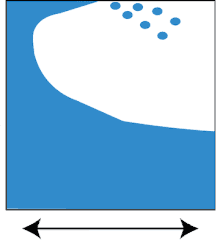Using the particle method, we can easily simulate the complex phenomenon (see movie 1). This is because the particle method expresses fluid as groups of particles. The particles are calculation points and are able to move flexibly. There are no difficulties with large deformation, splitting and coalescence of fluid. Simulations of water splashing are very easy for the particle method.

## Traditional methods for a Computational Fluid Dynamics Analsys (CFD) require meshing

We were taught in school - Meshing is the most important part in any of the computer simulations, because how you mesh can drastically change the results. This is why good meshing is very essential for a sound computer simulation to give good results. Mesh-Free Particle methods such as MPS have been devised as analytical methods to complement traditional mesh-based methods.

The lattice method such as finite difference method, finite element method, finite volume method, etc. uses a calculation lattice called a mesh to calculate physical quantities such as pressure and flow velocity. Mesh must be set beforehand including not only the area where the fluid exists but also the area where the fluid may flow in the initial state. The fluid flows from one mesh to the adjacent mesh, and at this time the inflow and outflow such as mass and momentum are calculated for each mesh. But, it is necessary to predict where the fluid flows and to create the mesh, and if the fluid flows in an unexpected direction, calculation cannot be performed unless the mesh is defined. Also, unnecessary calculations will occur if there is a mesh where fluid does not flow. Mesh generation is easy if the analysis area is simple shape, but meshing to areas of complicated shape such as automobile gearbox, air conditioning facility heat exchanger, etc. requires a lot of setup hours and analysis hours.

Since there is mesh dependency to some extent in fluid analysis, it is necessary to set mesh arrangement and coarse / fine in consideration of flow direction, flow velocity distribution and the like, and in order to perform highly accurate calculation, we have abundant knowledge and experience Is required. In the analysis involving a large deformation of the mesh, the mesh collapses and the calculation may end abnormally.

## Introducing Particleworks

The particle method models the fluid itself with particles without using a lattice that represents space. To be precise, "particle" of the particle method does not represent a substance such as water droplet but "calculation point" for calculating the flow velocity and pressure, which corresponds to the lattice point of the lattice method. Since the particles themselves represent the fluid flow, it is not necessary to set the analysis area in advance. This is very effective when tracking the state where droplets of fluid widely scatter. Free surface is also intuitively visualized by particle distribution. Even for containers and pipes of complicated shape, if there is CAD data of the wall shape, it is easy to model the fluid part. The analyst specifies only the initial particle spacing and the particles are evenly distributed according to the specified interval. This dramatically shortens the process of preparing an analysis model and enables analysts to concentrate on analysis and results verification.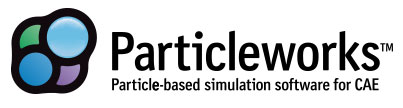Particleworks is an advanced CFD Software solution developed by Prometech, based on the Moving Particle Simulation (MPS) method.

For CFD Users, Particleworks can help you achieve faster, better process with reduced resource comsumption.

For Engineers relying on tests because traditional CFD is taking too long, complex and resource demanding. Particleworks can help you save money through CAE simulation.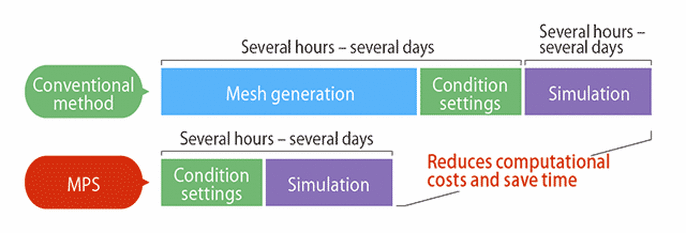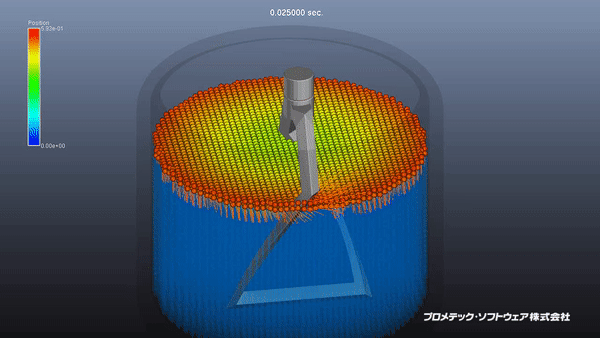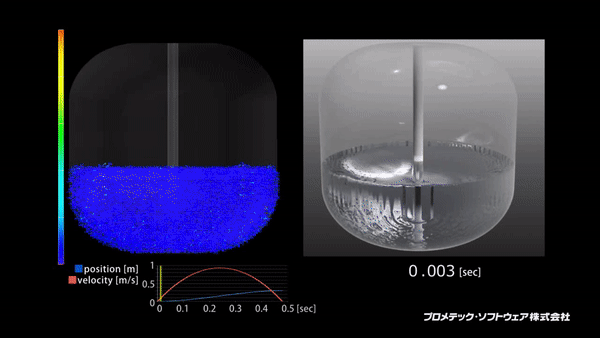## Benefits of Particleworks

• Gain significant time and cost savings with the Mesh-free particle method
• Achieve improved accuracy of free surface flow
• Overcome complex boundary geometries with flexible boundary conditions
• Accelerate flexible fluid power/fluid rigid body integrations
• Understand the modularity of chemical reactions and mixing